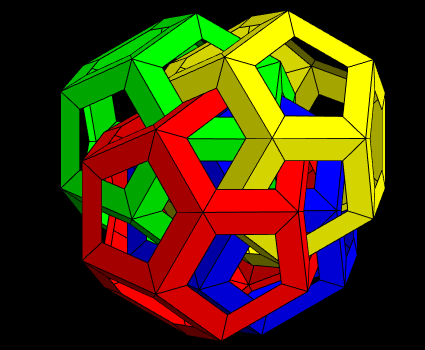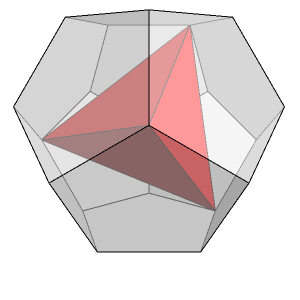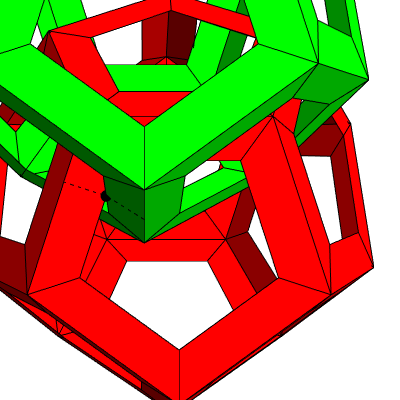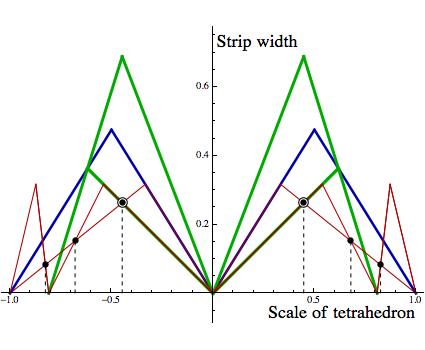# Optimised Origami

## by Greg EganIn a recent discussion on the mathematics of origami, John Baez raised a puzzle concerning a photograph of several interlocking origami dodecahedra, created by Dirk Eisner and included on the site Mathematical Origami. It was unclear from the photograph exactly how many dodecahedra the whole model contained (perhaps some were hidden from the camera, in the particular view shown), so the puzzle was: assuming the most symmetrical configuration, how many dodecahedra were present?John conjectured that the number was four, and that their centres were arranged at the vertices of a regular tetrahedron. This seemed plausible, especially since it’s possible to find sets of four vertices of a regular dodecahedron that are also vertices of a regular tetrahedron, as in the image on the right. The idea would be that you take a dodecahedron, create four congruent versions of it, then translate their centres to lie on the vertices of a regular tetrahedron (without rotating the dodecahedra at all). The actual size of the tetrahedron used to position the centres would be a parameter you would choose to make everything fit nicely, but its orientation would match that of one of the regular tetrahedra that can be found among the vertices of the original dodecahedron.

When I first tried to test this conjecture, I somehow managed to convince myself that it wouldn’t work: that whatever size you chose for the tetrahedron (so long as the dodecahedra were still close enough together to be mutually interlocked), this orientation would cause some of the origami strips to intersect. In fact I was completely wrong about that! I must have made a mistake in my initial calculations. However, my mistake prompted me to consider a related question: of all the ways you could arrange the centres of four (mutually interlocking) dodecahedra, which configuration would allow the origami strips to be as wide as possible without intersecting. Surely such an optimal configuration would also be highly symmetrical. It was only when I searched millions of configurations looking for the best one that I discovered my mistake ... because the best one I found was a regular tetrahedron, and it was oriented in exactly the same way as one of the tetrahedra formed from the dodecahedron’s vertices.The purpose of this page is to sketch the way an optimum configuration of the dodecahedra can be determined. In everything that follows, we won’t care about the pragmatic issues of how the dodecahedra can be held in place in a given configuration (whether it would be physically stable under gravity, etc.) What we wish to know is simply the placement of the centres of four mutually interlocking dodecahedra that would allow the widest strips, on purely geometrical grounds.

There are two ways that a configuration of the dodecahedra can impose an upper bound on the width of the strips. In the image on the left, a true edge of the green dodecahedron pierces one of the red dodecahedron’s faces in such a way that if the strips grew any larger, a red strip would enclose the piercing point (the black dot). One of the two green strips shared by the true edge also runs along the inner red edge, but that doesn’t change anything: the maximum strip width is set by the distance of the piercing point from the true edge of the red dodecahedron, indicated by the dashed line.

The length of that dashed line is a linear function of the three-dimensional vector by which the centre of the green dodecahedron is displaced from the centre of the red one. This function can be determined by some basic geometry, and its details will depend on (a) the choice of edge in the green dodecahedron, (b) the face of the red dodecahedron pierced by that green edge, and (c) which of the five edges of that red face lies closest to the piercing point. The choices for (b) and (c) can also be phrased as a choice of which of the 60 isosceles triangles that the 12 faces of the red dodecahedron can be divided into is pierced by the chosen green edge.

The value of this function will impose an upper bound on the strip width for a given configuration, but only when the displacement of the centres, which we’ll call δ, lies in the domain of relevance: the set of values for δ such that the chosen green edge actually does pierce the chosen red triangle. That set is easy to describe: it consists of a triangular prism whose six vertices are the translations that take either endpoint of the chosen edge to each of the three vertices of the chosen triangle.

Rather than piercing a triangle at a single point, an edge might end up lying in the plane of the triangle and intersecting it along an entire line segment. However, this will only happen in a two-dimensional subset of the space of displacements. In most cases, one of the edges sharing an endpoint with the one in question would be piercing the triangle (or a neighbouring triangle) in the normal way, and the functions we’ve already considered will bound the strip width appropriately. The only way that can fail to happen is if two true edges from the two dodecahedra are intersecting away from their endpoints, which is clearly not an optimal configuration. So we won’t include distance functions for these cases.The second way that a configuration can put a bound on the strip width is illustrated in the image on the right. Here, two inner edges touch, rather than a true edge and an inner edge. To deal with these cases, we can solve a set of equations in three variables: the strip width, and two variables describing the position along each inner edge of the point of contact.

Again, there are some “degenerate” cases. For example, if the two inner edges are parallel, they will meet along a whole line segment, rather than at a single point. But in that case, at the endpoints of the line segment either the same, or a more stringent bound would be set by the interaction between one of the original inner edges and an adjacent, non-parallel inner edge of the other dodecahedron. So if we assume that the two edges in these situations are not parallel, we will still obtain all the necessary bounds.

The distance shown by the two equal-length dashed lines in the diagram will be a linear function of the displacement of the centres, δ. The domain of relevance for that function will be bounded by planes in the three-dimensional space for δ where the point of contact lies on any of the six sides of the two isosceles triangles, but for the point of contact to lie on the true dodecahedron edge of one triangle it must also lie on the other, so the domain is a polyhedron with just five faces. The precise shape is a pyramid with a quadrilateral base: the four triangular faces consist of translations where the point of contact lies on each of the four non-true-edge sides of the intersecting triangles, and the base consists of translations where the point of contact lies on both true edges.

Now, given a collection of linear functions that put bounds on the strip width over their respective domains, we wish to find the configuration where the minimum of all the applicable bounds is as high as possible. Of course if we separated the dodecahedra completely there would be no bounds (except for the one imposed by the strips all meeting in the centre of each face), but we’re interested in the opposite extreme, where the displacements are small and all four dodecahedra overlap. To be precise, we will only consider displacements where an edge, if it pierces another dodecahedron’s face, does so in a displaced version of either of the two faces that share exactly one vertex with the edge.

If we impose no assumptions of symmetry, the full space for the relative displacements of the four centres is 9-dimensional: we can pick the translations from one centre to three of the others freely, and then the vectors between any of those three are fixed. Linear functions can only have extrema at the boundaries of their domains, but the quantity we want to maximise is the minimum of several linear functions, and the minimum switches between the functions in the collection whenever they are equal. So these points of equality are also potential extrema.

In order to pin down a single point in 9-dimensional space we need nine independent linear equations, which can be obtained by requiring 10 independent linear functions to take the same value. But we have a lot of linear functions to choose from: for each of the 12 pairings of the four dodecahedra we can consider the functions arising from 30 edges piercing any of 5 triangles in either of two faces. Then we have still more functions that arise from inner edges touching. Among the thousands that result from all these choices, there is some duplication, but the number of independent choices is still impractically high. The largest search of the 9-dimensional space I have conducted involved checking Binomial(44,10)=2,481,256,778 potential maxima. The 44 functions were chosen by examining a particular configuration of dodecahedra that resembled the photograph of the origami model under discussion, and assuming that the relevant bounds would come from functions where the same edges as in the model pierced either the same triangles, or neighbouring ones.

The result of checking those 2,481,256,778 configurations was that the one yielding the largest strip width had the four centres located at the vertices of a regular tetrahedron, aligned in exactly the same way as a regular tetrahedron formed by four vertices of the original dodecahedron. So — without claiming any kind of rigorous proof that this form of symmetry gives the best result — we will consider a simpler question: if we choose to place the four centres at the vertices of such a tetrahedron, and vary the tetrahedron’s overall size, what is the largest possible width for the origami strips?

This reduces the problem to one dimension, so instead of needing to check sets of 10 functions at a time, we only need to consider pairs. What’s more, the symmetry we’ve imposed collapses many more of the functions together, since it no longer makes any difference which pair of dodecahedra we’re talking about. Any pair could now be superimposed on any other, by means of a suitable rotation and translation.

As we vary the scale of the tetrahedron, multiplying one that we found among the dodecahedron’s vertices by a factor of s, the paths traced out by each of the three-dimensional displacements between dodecahedra will generally intersect the triangular prisms and pyramids discussed earlier along finite intervals, and the linear functions of s will have these intervals as their domains. But due to the high degree of symmetry, some paths will have glancing intersections with the three-dimensional domains, giving the new functions single points as their domains.

To find the desired optimum, we will check all endpoints of domains, and all points of intersection between pairs of functions. At each of these points, we need to calculate all the applicable bounds, and note the lowest. The highest value of that minimum across all points is the maximum strip width, and the value of s where it occurs tells us the optimum size for the tetrahedron.In the plot above, green lines indicate bounds on the strip width due to true edges of the dodecahedron, red lines indicate bounds due to inner edges, and blue lines are used for cases where identical functions and domains arise from both constraints. Where the functions are the same but the domains merely overlap, lines of different colour are superimposed.

It’s easy to see that the highest value for the minimum occurs when s ≈ ±0.45 and the strip width ≈ 0.26. The exact values are:

scale factor = 1/√5 ≈ 0.447214
distance between centres = √[(3 + √5)/5] ≈ 1.02333
maximum strip width = √[(1 – 1/√5)/8] ≈ 0.262866

Here the strip width and the distance between the dodecahedron’s centres are expressed in units where the edge length of the dodecahedron is 1. The scale factor s is relative to a tetrahedron whose four vertices are a subset of the dodecahedron vertices.Science Notes / Optimised Origami / created Sunday, 8 December 2013